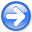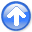Efficient depth extrapolation of waves in elastic isotropic media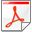Next: Implementation and Results Up: Maharramov: Efficient elastic modeling Previous: Introduction

# The Method

We start with the wave equation governing the displacement in an arbitrary heterogenous isotropic elastic medium in the Navier form (see (Segall, 2010)):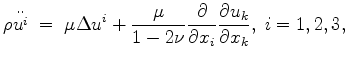(1)

wheredenote the components of a displacement field,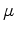is the shear modulus,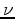is Poisson's ratio for the medium, and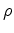is the density. In this paper we consider a heterogenous elastic medium under the assumption of local homogeneity - otherwise the elastic moduli would not be factored outside of the differentiation operators in equation 1. However, our method can be extended to cover the case when the local homogeneity assumption is dropped. Freezing'' the coefficients of equation 1 and applying the Fourier transform in time and horizontal variables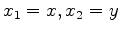, and substituting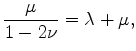(2)

where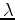is the Lamé coefficient (see (Mavko et al., 2009),(Segall, 2010)), we get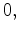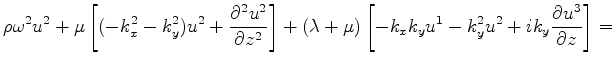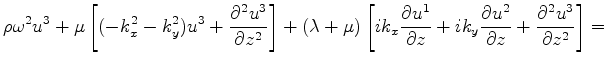(3)

where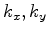are horizontal wave numbers and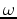is the frequency. The left-hand side of system 3 is the result of an ordinary differential operator applied to a vector-functionand parameterized by horizontal wave numbers. In the present form equations 3 cannot be used for computationally efficient explicit depth extrapolation in a heterogeneous medium; however, these equations can be used for modeling displacements by solving a series of boundary-value problems (see (Maharramov, 2012)). In (Maharramov, 2012) it was suggested that equations 3 might be factorized in such a way as to allow solving them by alternating one-way extrapolation in opposite directions. More specifically, we seek a factorization of operator equation 3 of the form: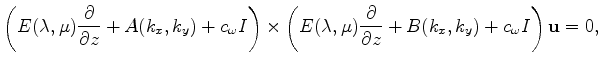(4)

where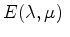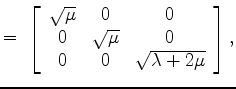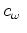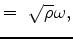(5)

andare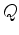matrices with components that are complex-valued functions of the horizontal wave numbers,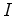is theidentity matrix. Performing the multiplication in equation 4 and using equation 3, we obtain: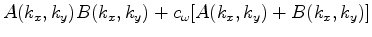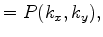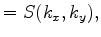(6)

where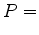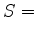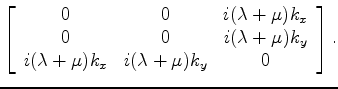(7)

Combining equations 6 and 7, we get the following equation for the operators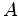and: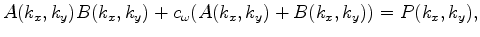(8)

where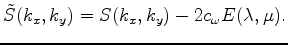(9)

Equations 4, 8 in combination with equations 7 and 9 suggest the following procedure for extrapolating solutions to system 1 in depth:
1. Solve the system of matrix equations 8 for, for each pair of horizontal wave numbersand two reference values of each elastic parameterand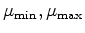;
2. Evaluate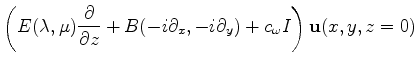from the initial conditions and assign the value to an auxiliary function;
3. Solve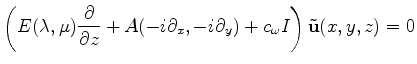(10)

by downward continuing in depth, using the formula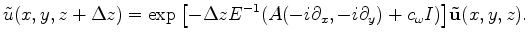(11)

4. Perform each step of the depth extrapolation for four combinations of the reference elastic parameters, then apply the inverse Fourier transform to the four fields and interpolate at each spatial point of the depth slice using true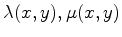as e.g. in the PSPI method (see (Biondi, 2005)).
5. After reaching the desired maximum depth, find the solution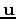by upward extrapolation: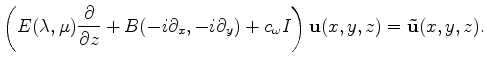(12)

6. Repeat the above steps for each frequency component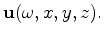The above algorithm is stable if the spectrum of matrix(13)

is not in the interior of the left half-plane, and the spectrum of(14)

is not in the interior of the right half-plane. While the above algorithm tries to mimic two-way wave propagation, it is effectively just an approximation to the propagation process as it ignores the interaction between the up and down-going wave at intermediate depth steps. A less accurate alternative would be to downward-continue the wave field using equation 11 in a way similar to the one-way depth extrapolation using the scalar square-root equation (see (Claerbout, 1985),(Biondi, 2005)). The latter approach would be unable to image any dips beyond 90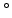, however, it would reduce the cost of extrapolation by a further factor of 2. Note the cost of solving equation 10 in depth is roughly three times that of solving the scalar square-root equation.

The above analysis may be extended to the case of an arbitrary anisotropic elastic medium. The fact that the components of the pseudo-differential operator matrices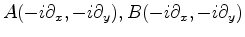are not given in an analytical form, but are only computed numerically, does not limit their applicability.

Factorization of system 3 in the elastostatic case was one of the approaches mentioned by the author in Maharramov (2012). However, the one-way extrapolation technique is mostly useful for elastodynamic problems as the passband of the factorized depth extrapolators (e.g., as in equation 11) narrows down to zero with the temporal frequency passing to the zero static limit.

maximageigenval
Figure 1.
The phase of a phase-shift operator corresponding to the maximum imaginary part of the eigenvalues of operator 15. Multicomponent phase-shift'' is defined by three such scalar phase-shift operators and amatrix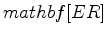of equation 16.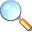Note that equation 1 uses elastic parameterization that degenerates into a singularity if the shear modulus is equal to zero. This is not causing any problems with purely acoustic wave extrapolation as the singularity is effectively removed from equations 3 by the substitution in equation 2.Efficient depth extrapolation of waves in elastic isotropic mediaNext: Implementation and Results Up: Maharramov: Efficient elastic modeling Previous: Introduction

2012-10-29